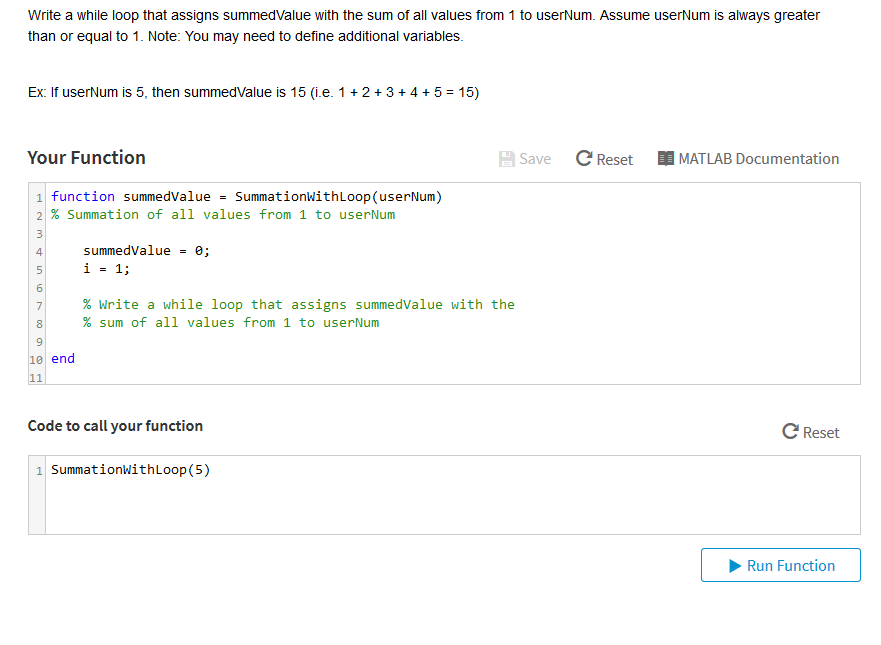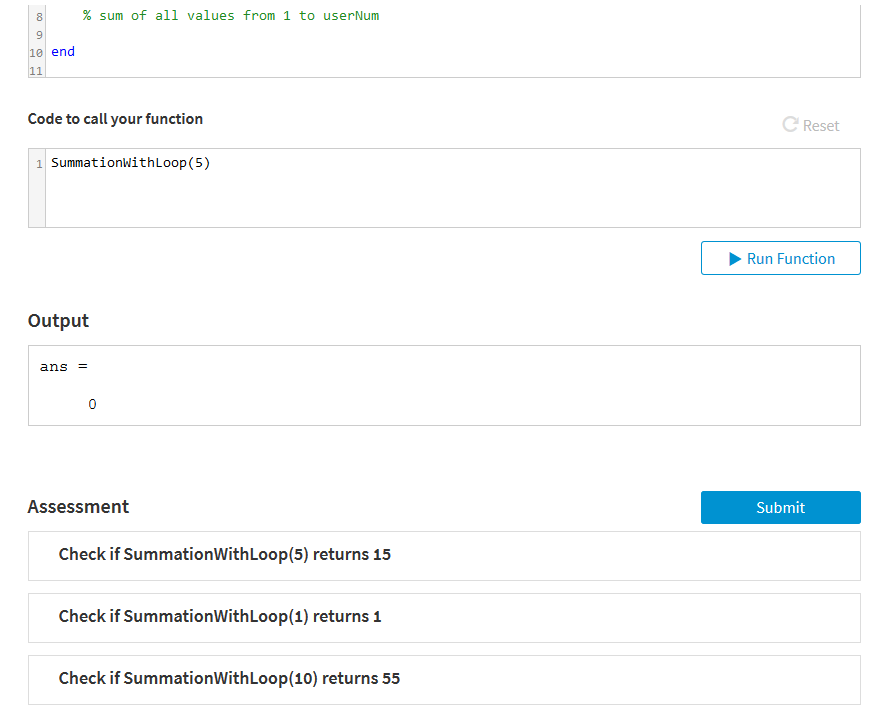# Question & Answer: Use the Programming Language —- > MATLAB…..

Use the Programming Language —- > MATLAB <—Don't use plagiarized sources. Get Your Custom Essay on
Question & Answer: Use the Programming Language —- > MATLAB…..
GET AN ESSAY WRITTEN FOR YOU FROM AS LOW AS \$13/PAGE

Write a while loop that assigns summedValue with the sum of all values from 1 to userNum. Assume userNum is always greater than or equal to 1. Note: You may need to define additional variables. Ex. If userNurm is 5, then summedalue is 15(ie 1+2+3+4+5=15) Your Function a Save Reset MATLAB Documentation 1 function summedValue = SummationWithLoopCuserNum) 2% summation of all values from 1 to userNum summedValue = e; i-1; 4 5 6 % Write a while loop that assigns summedValue with the % sum of all values from 1 to userNum 8 9 e end Code to call your function C Reset 1 SummationwithLoop (5) Run Function

While loop code in the given Matlab question is:-
while( i <= userNum )
summedValue = summedValue + i;
i = i + 1;
end

Yes below are the functions which returns value as mentioned.
SummationWithLoop(5) returns 15
SummationWithLoop(1) returns 1
SummationWithLoop(10) returns 55

Here is the code i have checked to verify the same and also attached screenshot for your reference.

function summedValue = SummationWithLoop(userNum)
i = 1;
summedValue = 0;
% while loop execution
while( i <= userNum )
summedValue = summedValue + i;
i = i + 1;
end
fprintf(‘Value of Sum for given number %d is %d.n’, userNum, summedValue);
end

SummationWithLoop(5);
SummationWithLoop(1);
SummationWithLoop(10);

Code output screenshot:
https://pasteboard.co/GFrWA0M.png